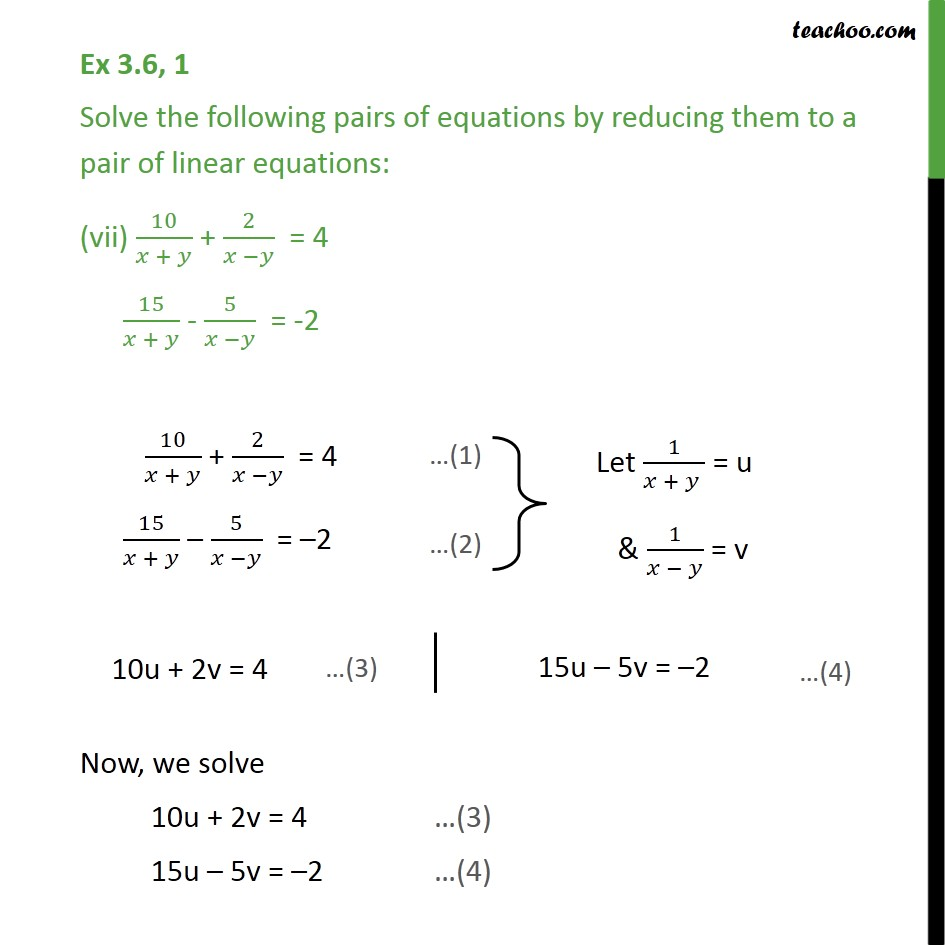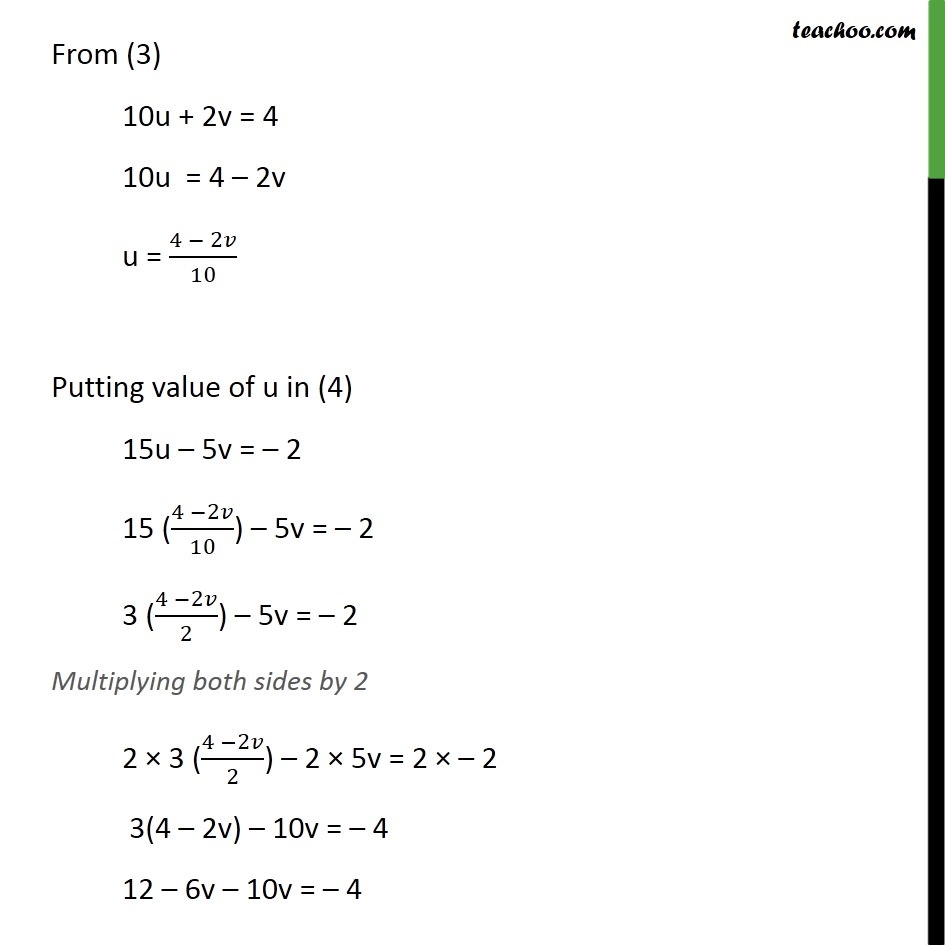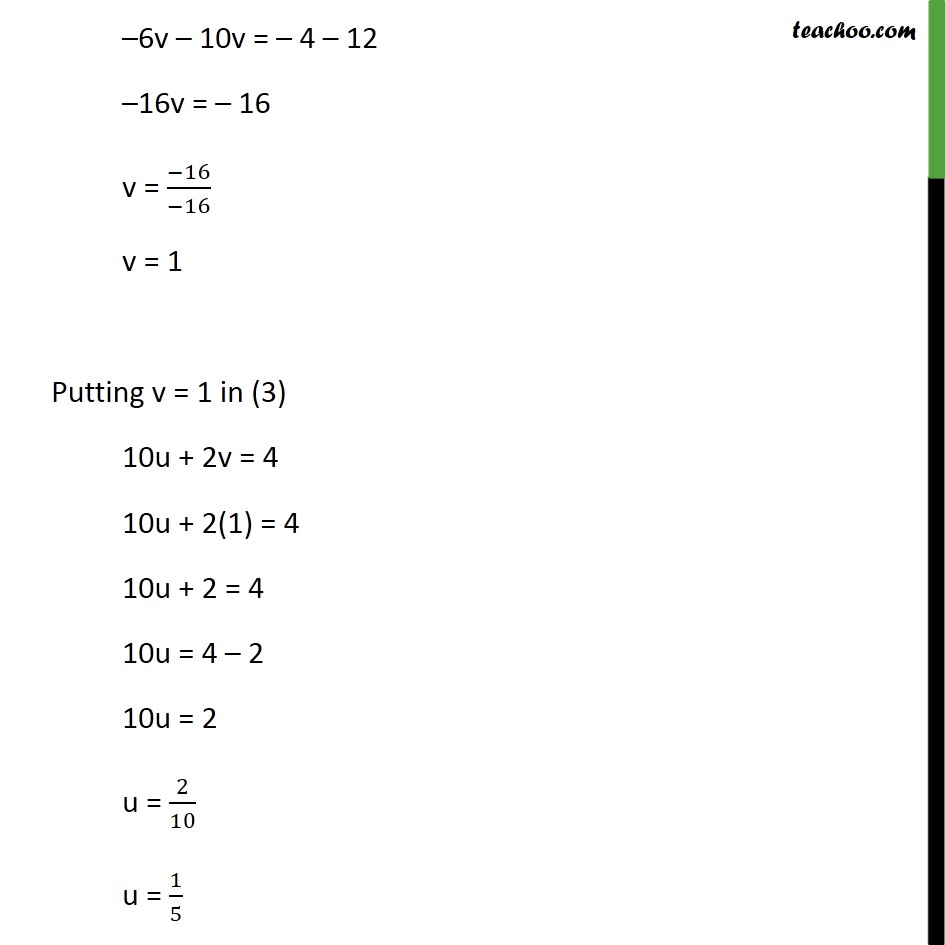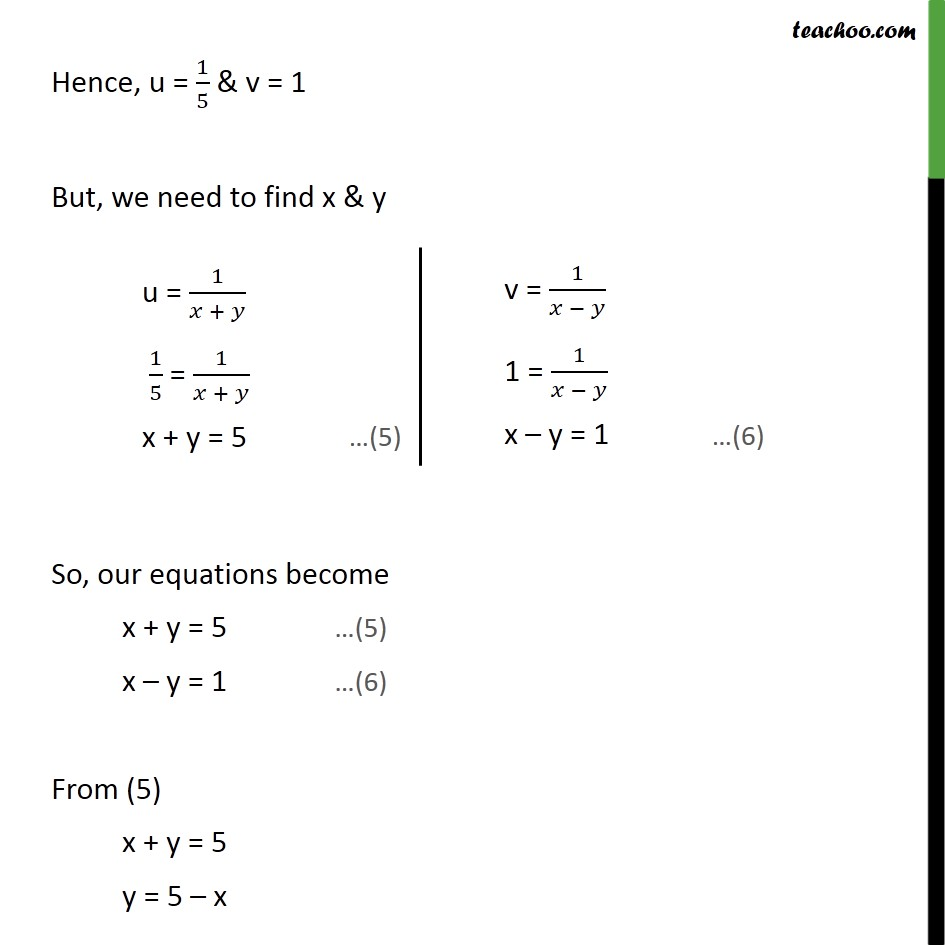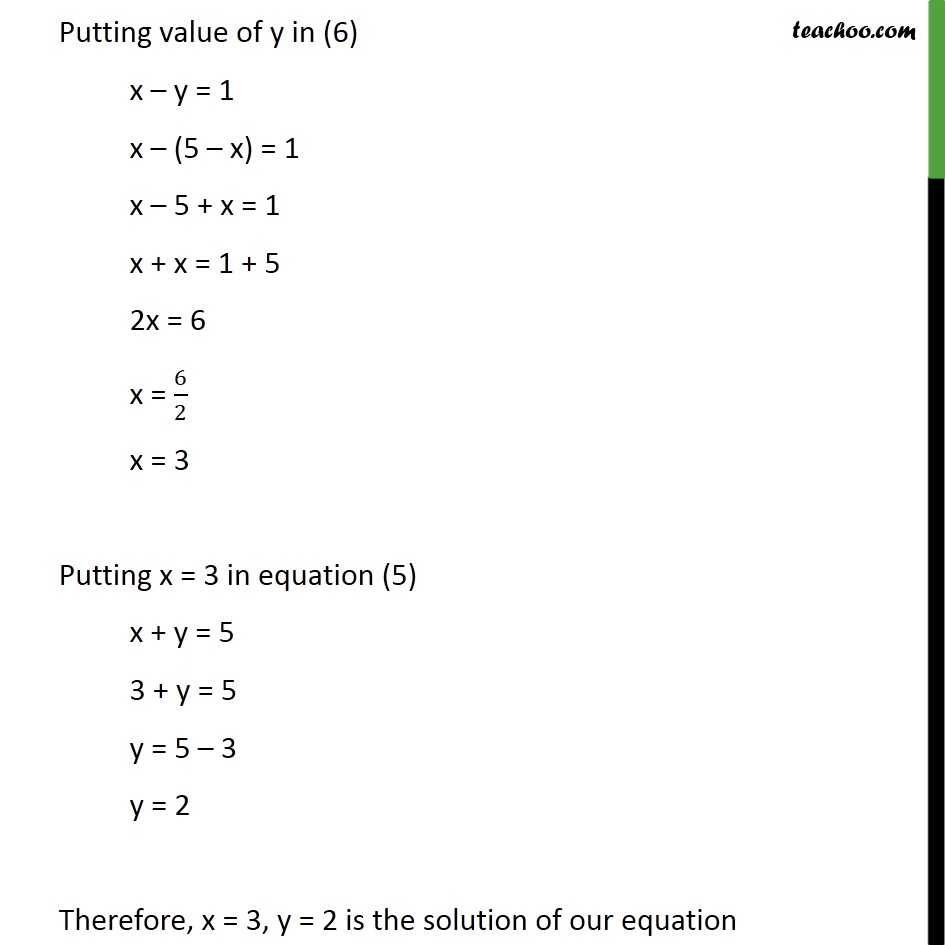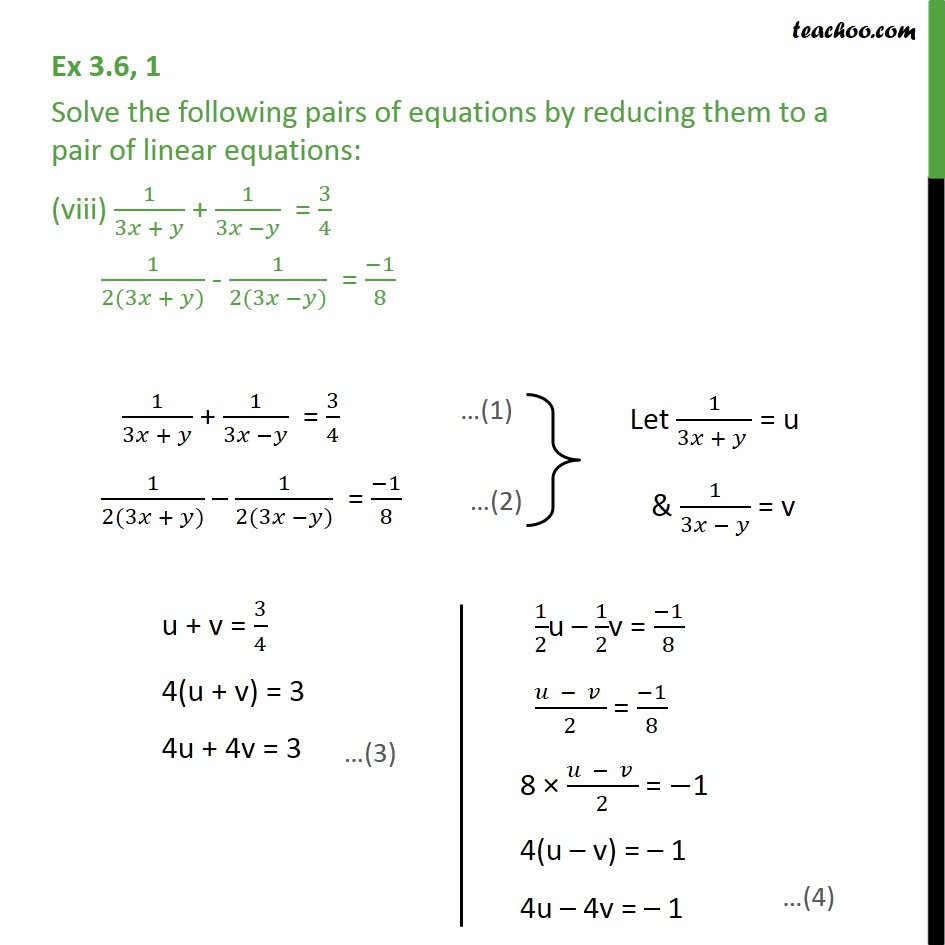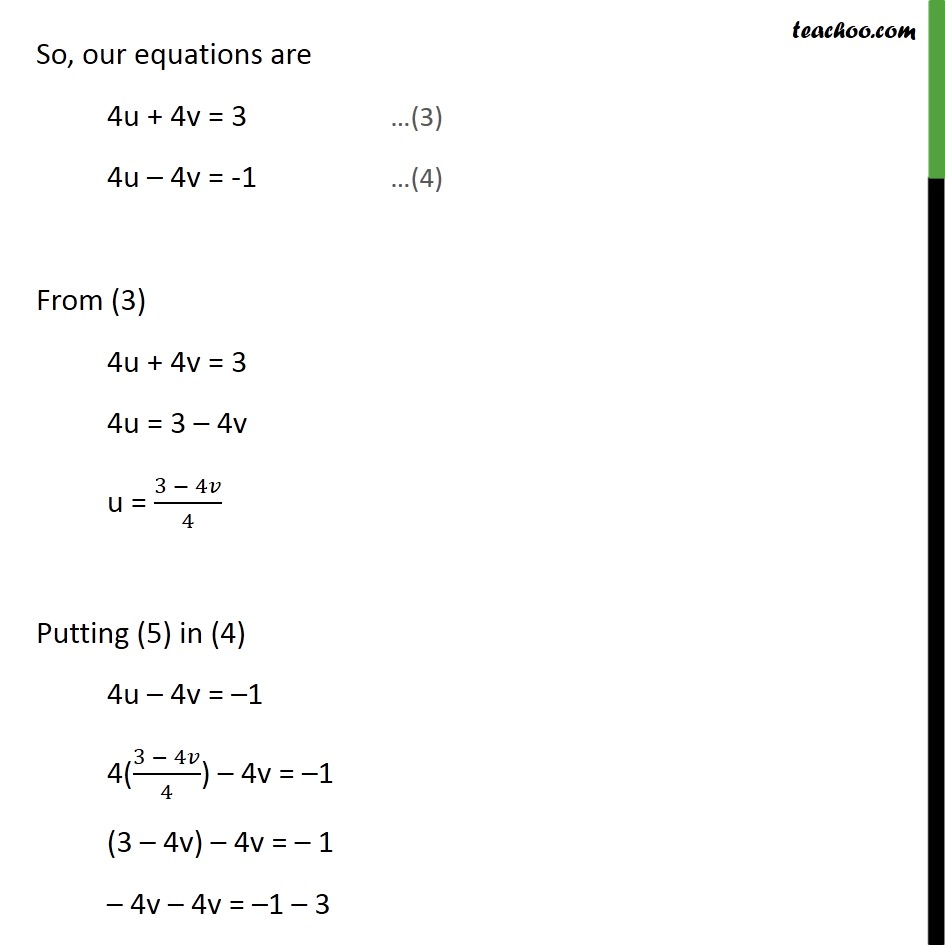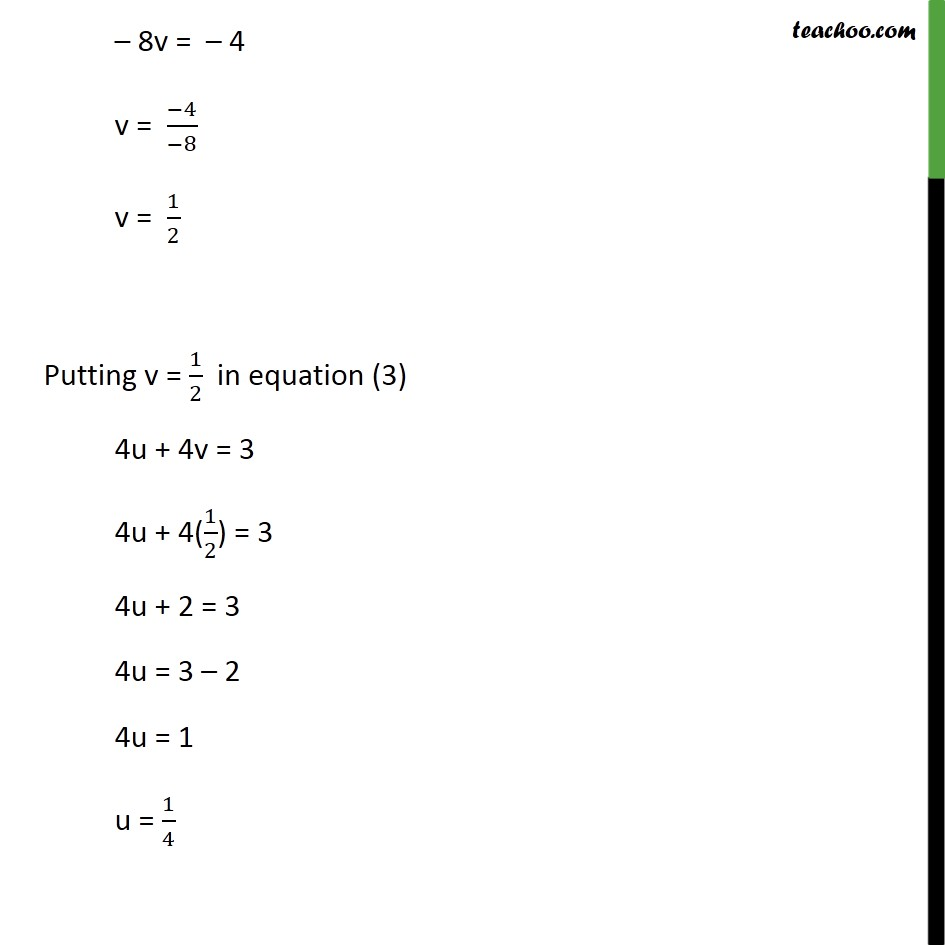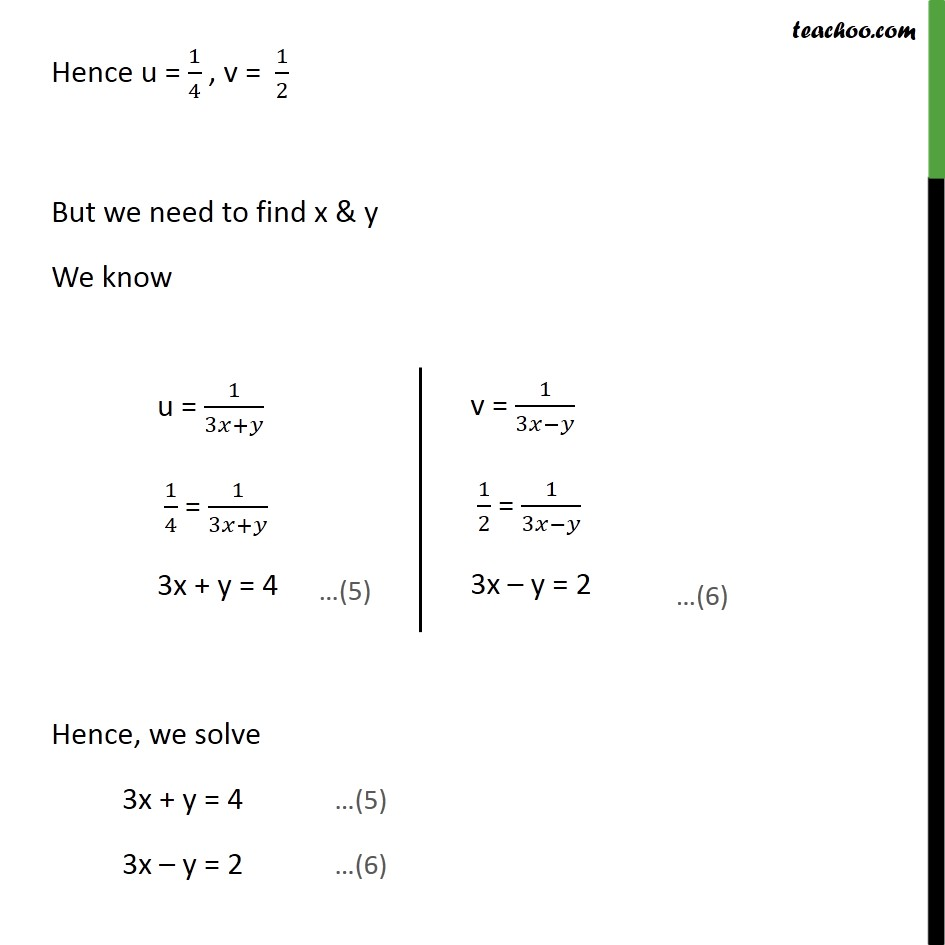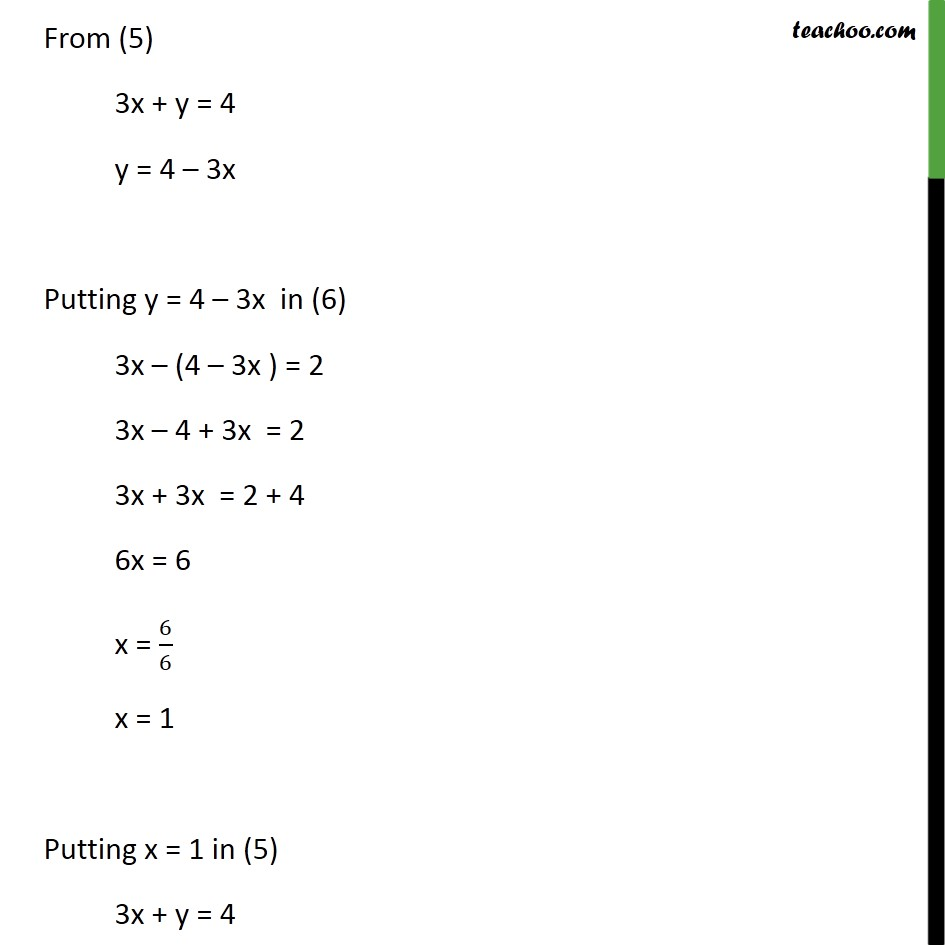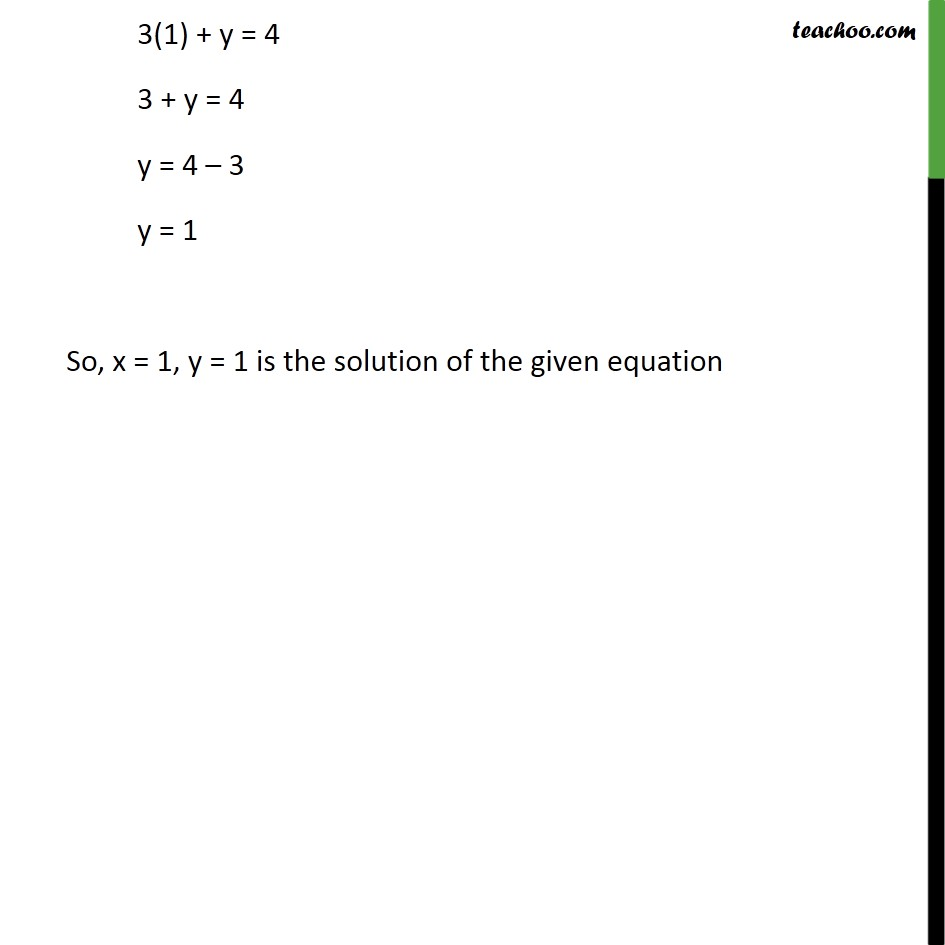1. Class 10
2. Important Questions for Exam - Class 10
3. Chapter 3 Class 10 Pair of Linear Equations in Two Variables

Transcript

Ex 3.6, 1 Solve the following pairs of equations by reducing them to a pair of linear equations: (vii) 10/(x + y) + 2/(x − y) = 4 15/(x + y) - 5/(x − y) = -2 10/(x + y) + 2/(𝑥 − y) = 4 15/(x + y) – 5/(𝑥 − y) = –2 Now, we solve 10u + 2v = 4 …(3) 15u – 5v = –2 …(4) From (3) 10u + 2v = 4 10u = 4 – 2v u = (4 − 2v)/10 Putting value of u in (4) 15u – 5v = – 2 15 ((4 −2v)/10) – 5v = – 2 3 ((4 −2v)/2) – 5v = – 2 Multiplying both sides by 2 2 × 3 ((4 −2v)/2) – 2 × 5v = 2 × – 2 3(4 – 2v) – 10v = – 4 12 – 6v – 10v = – 4 –6v – 10v = – 4 – 12 –16v = – 16 v = (−16)/(−16) v = 1 Putting v = 1 in (3) 10u + 2v = 4 10u + 2(1) = 4 10u + 2 = 4 10u = 4 – 2 10u = 2 u = 2/10 u = 1/5 Hence, u = 1/5 & v = 1 But, we need to find x & y So, our equations become x + y = 5 …(5) x – y = 1 …(6) From (5) x + y = 5 y = 5 – x Putting value of y in (6) x – y = 1 x – (5 – x) = 1 x – 5 + x = 1 x + x = 1 + 5 2x = 6 x = 6/2 x = 3 Putting x = 3 in equation (5) x + y = 5 3 + y = 5 y = 5 – 3 y = 2 Therefore, x = 3, y = 2 is the solution of our equation Ex 3.6, 1 Solve the following pairs of equations by reducing them to a pair of linear equations: (viii) 1/(3𝑥 + 𝑦) + 1/(3𝑥 −𝑦) = 3/4 1/(2(3𝑥 + 𝑦)) - 1/(2(3𝑥 −𝑦)) = (−1)/8 1/(3𝑥 + 𝑦) + 1/(3𝑥 −𝑦) = 3/4 1/(2(3𝑥 + 𝑦)) – 1/(2(3𝑥 −𝑦)) = (−1)/8 So, our equations are 4u + 4v = 3 …(3) 4u – 4v = -1 …(4) From (3) 4u + 4v = 3 4u = 3 – 4v u = (3 − 4𝑣)/4 Putting (5) in (4) 4u – 4v = –1 4((3 − 4𝑣)/4) – 4v = –1 (3 – 4v) – 4v = – 1 – 4v – 4v = –1 – 3 – 8v = – 4 v = (−4)/(−8) v = 1/2 Putting v = 1/2 in equation (3) 4u + 4v = 3 4u + 4(1/2) = 3 4u + 2 = 3 4u = 3 – 2 4u = 1 u = 1/4 Hence u = 1/4 , v = 1/2 But we need to find x & y We know Hence, we solve 3x + y = 4 …(5) 3x – y = 2 …(6) From (5) 3x + y = 4 y = 4 – 3x Putting y = 4 – 3x in (6) 3x – (4 – 3x ) = 2 3x – 4 + 3x = 2 3x + 3x = 2 + 4 6x = 6 x = 6/6 x = 1 Putting x = 1 in (5) 3x + y = 4 3(1) + y = 4 3 + y = 4 y = 4 – 3 y = 1 So, x = 1, y = 1 is the solution of the given equation

Chapter 3 Class 10 Pair of Linear Equations in Two Variables

Class 10
Important Questions for Exam - Class 10

About the AuthorDavneet Singh
Davneet Singh is a graduate from Indian Institute of Technology, Kanpur. He has been teaching from the past 9 years. He provides courses for Maths and Science at Teachoo.